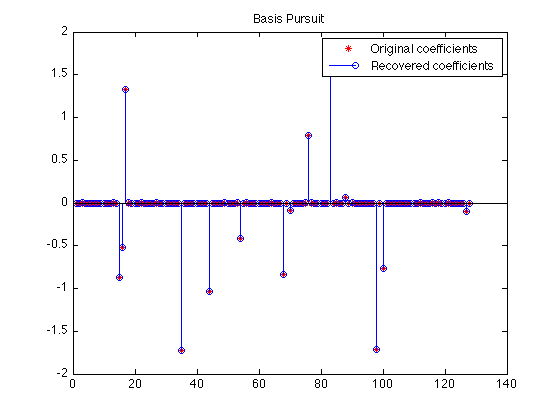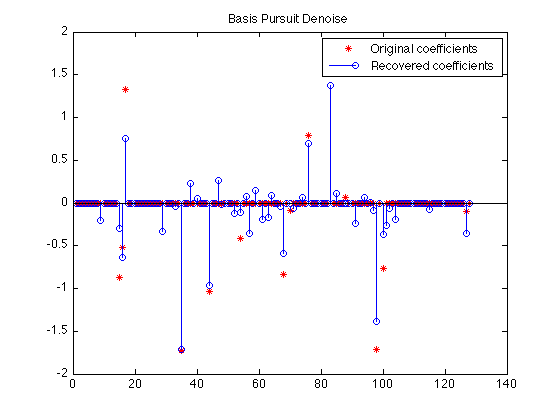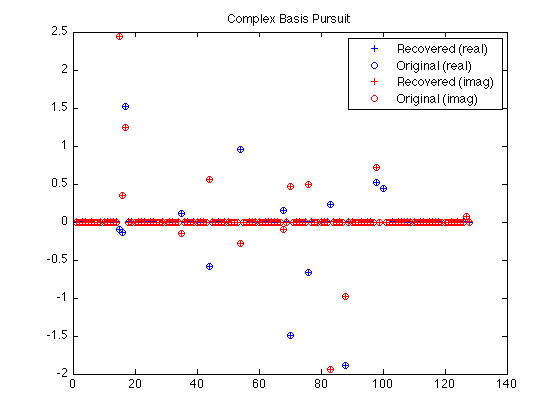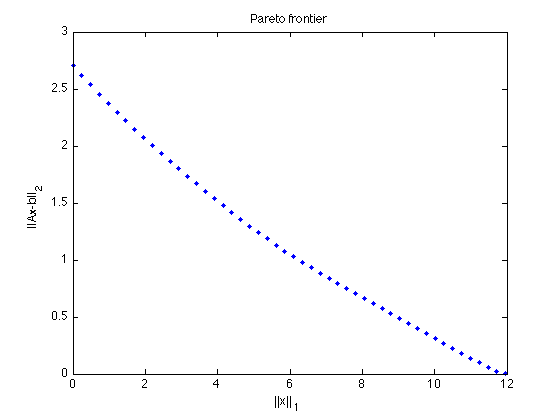# SPGL1: A Brief Tour

Michael P. Friedlander, June 2008

SPGL1 is a solver for one-norm regularized least-squares. It is suitable for problems that are large-scale and in the complex domain. This document gives a brief tour of SPGL1's main features.

## Generate random data

The examples below are based on randomly generated data. We'll begin by creating a random m-by-n encoding matrix and a sparse solution vector:

```rand('twister',0); randn('state',0);
m = 50; n = 128; k = 14;                   % No. of rows (m), columns (n), and nonzeros (k)
[A,Rtmp] = qr(randn(n,m),0);               % Random encoding matrix with orthogonal rows
A  = A';                                   % ... A is m-by-n
p  = randperm(n); p = p(1:k);              % Location of k nonzeros in x
x0 = zeros(n,1); x0(p) = randn(k,1);       % The k-sparse solution
b  = A*x0;                                 % The right-hand side corresponding to x0
```

## Basis pursuit

The basis pursuit (BP) problem

`  minimize ||x||_1  subject to  Ax = b`

often finds the sparsest solution to the linear system of equations Ax = b. The call to SPGL1 is straightforward:

```opts = spgSetParms('verbosity',0);         % Turn off the SPGL1 log output
x = spg_bp(A, b, opts);
```

Let's verify that the true sparse solution was recovered correctly:

```plot(x0,'r*'); hold on
stem(x ,'b '); hold off
legend('Original coefficients','Recovered coefficients');
title('Basis Pursuit');
```As a final check, the residual should be nearly zero:

```norm(b - A*x)
```
```ans =
8.3531e-05
```

## Basis pursuit denoise

If there was noise inherent in the signal that we measured, it would be better to instead solve the basis pursuit denoise (BPDN) problem

`  minimize ||x||_1  subject to  ||Ax - b||_2 <= sigma,`

which only approximately fits the equation Ax=b. In this experiment, we'll set sigma = 0.1:

```b = A * x0 + randn(m,1) * 0.075;
sigma = 0.1;                                     % Desired ||Ax - b||_2
opts = spgSetParms('verbosity',0);
x = spg_bpdn(A, b, sigma, opts);
```

We don't expect the recovery to be exact this time because of the noise:

```plot(x0,'r*'); hold on
stem(x ,'b '); hold off
legend('Original coefficients','Recovered coefficients');
title('Basis Pursuit Denoise');
```The corresponding residual should have a norm (nearly) equal to sigma (0.1):

```norm(b - A*x)
```
```ans =
0.0999
```

## LASSO

The Lasso problem puts a constraint directly on the one-norm of the solution:

`  minimize ||Ax - b||_2  subject to  ||x||_1 <= tau`

Here, we'll recover a least-squares solution with a one-norm equal to pi -- an easily recognizable number! We'll turn on SPGL1's output so that we can see it's progress:

```opts = spgSetParms('verbosity',1);
tau = pi;
x = spg_lasso(A, b, tau, opts);
```
``` ================================================================================
SPGL1  v. 1017  (Mon, 16 Jun 2008)
================================================================================
No. rows              :       50      No. columns           :      128
Initial tau           : 3.14e+00      Two-norm of b         : 2.61e+00
Optimality tol        : 1.00e-04      Target one-norm of x  : 3.14e+00
Basis pursuit tol     : 1.00e-06      Maximum iterations    :      500

Iter      Objective   Relative Gap      gNorm   stepG    nnzX    nnzG
0  2.6116134e+00  8.8203906e-01   9.57e-01     0.0       0       0
1  1.7351041e+00  1.5596782e-01   5.16e-01     0.0      10       1
2  1.7035044e+00  3.5470990e-02   4.43e-01     0.0       6       1
3  1.6985890e+00  1.0356556e-02   4.16e-01     0.0       6       1
4  1.6984830e+00  1.3137969e-03   4.09e-01     0.0       6       3
5  1.6984820e+00  3.5582204e-04   4.09e-01     0.0       6       6
6  1.6984819e+00  2.5425067e-05   4.09e-01     0.0       6       6

EXIT -- Optimal solution found

Products with A     :       7        Total time   (secs) :     0.0
Products with A'    :       7        Project time (secs) :     0.0
Newton iterations   :       0        Mat-vec time (secs) :     0.0
Line search its     :       0        Subspace iterations :       0

```

The one-norm of the solution:

```norm(x,1)
```
```ans =
3.1416
```

## Complex variables

Many signal-processing problems involve complex-valued coefficients. In this example, we create a solution that is sparse in the Fourier domain. We simulate missing data by randomly restricting the rows of the Fourier operator. The resulting BP problem is

` minimize  ||z||_1  subject to  R*F*z = R*b,`

where R is a restriction operator and F is a Fourier operator. Keep in mind that z is a complex variable, and that

` ||z||_1 = sum_j ( sqrt( x_j^2 + y_j^2 ) ), where z_j = x_j + i*y_j.`

We use the partial Fourier operator

` z = partialFourier(idx,n,x,mode);`

the index set idx

```idx = randperm(n); idx = idx(1:m);
```

determines which rows of the Fourier operator are kept. We create an operator that conforms to SPGL1's requirements:

```opA = @(x,mode) partialFourier(idx,n,x,mode);  % This is now "A"
```

Create sparse coefficients and the right-hand side b:

```z0 = zeros(n,1);
z0(p) = randn(k,1) + sqrt(-1) * randn(k,1);
Rb = opA(z0,1);                                 % Rb = R*A*z0
```

Fire up SPG_BP in the usual way. Note that in this, case, the first argument is a function:

```opts = spgSetParms('verbosity',0);
z = spg_bp(opA,Rb,opts);
```

Plot the recovered and original coefficients:

```plot(1:n,real(z),'b+',1:n,real(z0),'bo', ...
1:n,imag(z),'r+',1:n,imag(z0),'ro');
legend('Recovered (real)', 'Original (real)', ...
'Recovered (imag)', 'Original (imag)');
title('Complex Basis Pursuit');
```## The Pareto curve

The Pareto curve traces the optimal tradeoff between the one-norm of the solution and the two-norm of the residual. We take advantage here of SPGL1's warm-start capability and sample the Pareto curve at a bunch of points.

We do this by solving a sequence of LASSO problems for increasing values of tau. Larger values of tau will yield smaller residuals. In fact, if phi(tau) is the norm of the residual corresponding to a given tau, then phi is a continuously differentiable function [BergFriedlander08].

Because here we wish to warm-start SPGL1, we need to use its expert interface, rather than the simpler interface available via SPG_LASSO.

```b = A*x0;
tau = linspace(0,1.01*norm(x0,1),50);      % Values of tau at which to sample
phi = zeros(size(tau));                    % Preallocate the vector of residuals.
x = zeros(n,1);                            % Initialize the solution.
opts = spgSetParms('verbosity',0);
for i=1:length(tau)
[x,r] = spgl1(A,b,tau(i),[],x,opts);   % Re-solve LASSO(tau) with a different tau.
phi(i) = norm(r,2);                    % SPGL1's 2nd output arg is the residual.
end
```

Plot the curve.

```plot(tau,phi,'b.');
title('Pareto frontier');
xlabel('||x||_1'); ylabel('||Ax-b||_2');
```## Reference

[BergFriedlander] E. van den Berg and M. P. Friedlander, "Probing the Pareto frontier for basis pursuit solutions", January 2008 (revised May 2008). To appear in SIAM Journal on Scientific Computing.

```% \$Id: spgexamples.m 1077 2008-08-20 06:15:16Z ewout78 \$
```